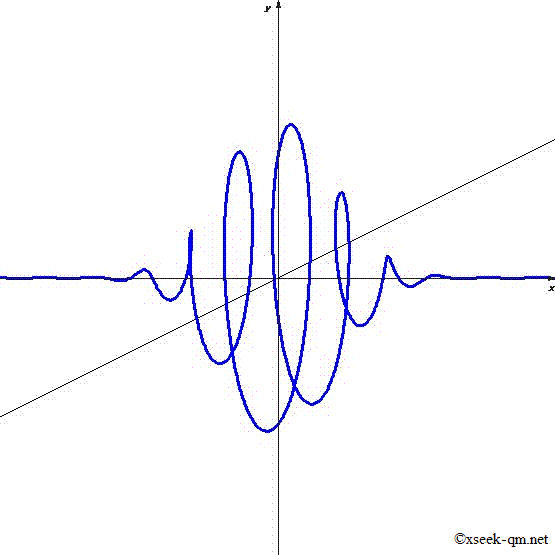# Mystery of wave function

2013/6/30
Published 2002/2/27

Wave function describes the probability of observing the particle. What is the physical meaning of the wave function? We would like to consider this topic.Wave function

## The shape of the wave function

For example, I suppose that one electron is flying. Did you imagine a flying ball of about 1 centimeter in diameter? We can describe the state of the electron by the wave function. We can express it in the above figure.

This figure represents the wave function of one-dimensional. Right direction is the direction of travel of the electron. An upward direction is an imaginary number. Oblique direction is the real number. In order to express the wave function we need three-dimensional space because it is a complex number. Many people might think that the complex number means an impossible thing. However, it is normal "helix" in the figure.

The distance from the horizontal axis is the height of the wave function. It is called the absolute value of the wave function. Direction from the horizontal axis is the angle of the wave function. It is called the phase of the wave function.

There might be people that do not have a mysterious impression about the picture. However, I believe that there are many people who have the following impression.

"This picture does not look like an electron at all."

I think the impression is a normal impression.

## Copenhagen interpretation

The radius of the electron is smaller than 1 femtometer. 1 femtometer is 10-15 meter. The radius of the nucleus is 1 femtometer. The electron spreads to the size of atoms. The radius of the atoms is 53,000 femtometers. If the nucleus is the ball with a radius of 1 millimeter, the electrons are spreading to the region of radius of 53 meters. We can find the electron in one place. It is called a wave function collapse.

I think it is a mysterious thing. Does the wave disappear suddenly when we observe? There is Copenhagen interpretation that is an idea describes this mystery. Copenhagen interpretation explains these phenomena as follows.

• Physical systems generally do not have definite properties prior to being measured.
• Quantum mechanics can only predict the probabilities that measurements will produce certain results.

The idea of the Copenhagen interpretation is very reasonable as this. However, based on my world-view, I have a mysterious impression about this idea. For example, it is the following feeling. "Though the iPhone was working properly, when I look at the interior, --- it was empty!"

## Physical meaning of the wave function

What is the physical meaning of the wave function? What does the wave function represent?

• The wave function continues far away to the world's end. However, why does the wave function discontinue in the middle?
• Even if we multiply the absolute value of the wave function by a constant, the calculated result does not change. However, what is the physical meaning of the absolute value of the wave function?
• Even if we rotate the phase of the wave function by a certain angle, the calculated result does not change. However, what is the physical meaning of the phase of the wave function?

We are living in a three-dimensional space. Einstein, told us that a space consists of the material that we are able to bend. Therefore, there is a possibility that the wave function also consists of the material that we are able to bend. The absolute value of the wave function might be something like the length of a space. The phase of the wave function might be something like the angle of space.

Related Articles: